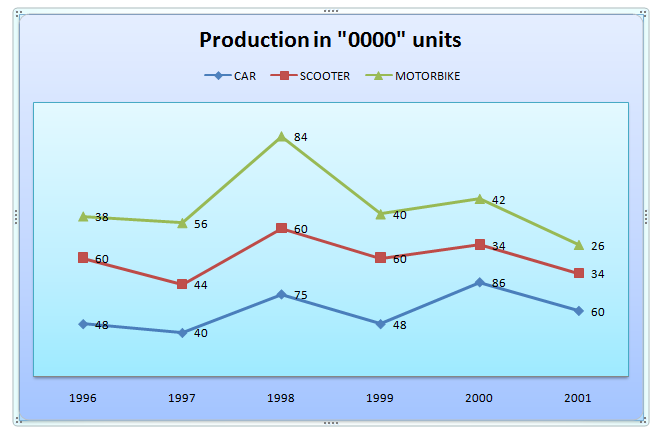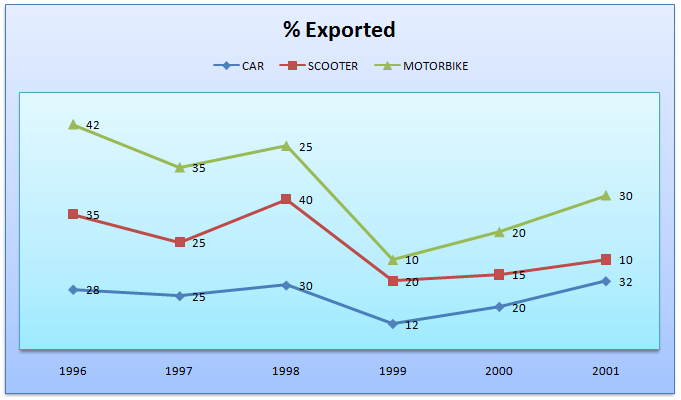Directions (Q. Nos. 1-5) Study the following line graphs which show the production (in ‘0000 units) and percentage export of scooters, motorbikes and car respectively over the years.Question 1: In which of the following years was the production of cars more than 50% of the total production?
(a) 2000
(b) 2001
(c) 1998
(d) 1996

Question 2. Find the total number of automobiles exported in the year 1999.
(a) 227600
(b) 207600
(c) 217600
(d) 220000

Question 3. Find the ratio of cars, scooters and motorbikes exported in 1996.
(a) 25 : 16 : 19
(b) 16 : 25 : 19
(c) 19 : 16 : 25
(d) 6 : 5 : 1

Question 4. If the ratio of export prices of a cars, scooter and motorbike was 2 : 1 : 1.5 in 1998, what was the proportion of their export earnings?
(a) 4 : 2 : 3
(b) 6 : 1 : 21
(c) 30: 16: 21
(d) Cannot be determined

Question 5. In which of the following years was the production of motorbikes exactly 40% of the total production of automobiles in that years?
(a) 1997
(b) 2000
(c) 1999
(d) 1996

Answer 1: (a) We have to check for those years where total value of production of scooter and bikes < value of car. By observation we can see that in the year 2000, the total production of cars was 860000 and the total production of scooters and bikes was 760000. Hence in 2000, the production of cars was more than 50% of the total production. Correct option is (a)

Answer 2: (c) We haveTotal= 57600 + 120000 + 40000 = 217600

Correct option is (c)

Answer 3: (b) We haveRatio: 134400 : 210000 : 159600 or 16: 25: 19.

Correct option is (b)

Answer 4: (c) We haveWe have The ratio of cars: scooters: motorbikes = 225000 :240000 : 210000 = 225:240:210 = 45:48:42 or 15: 16: 14

The ratio of export prices of cars: scooters: motorbikes = 2:1:1.5

The ratio of export earnings of cars: scooters: motorbikes = 15 × 2: 16 × 1: 14 × 1.5 = 30: 16: 21

Option (c) is correct.

Answer 5: (a)Keep in mind: You don’t need to calculate for all the years. Calculations should be done for the years which are given in the options, since, ultimately one of them is the answer. The years which are marked as bold in the column are only to be calculated.

It can be clearly observed that in 1997 the motorbike production is 560000 which is 40% of the total production. Therefore, (a) is the correct option.